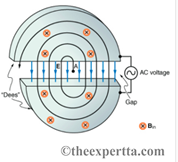# Problem: A cyclotron accelerates charged particles as shown in the figure. The magnetic field contains the particles within the machine while the electric field in the gap accelerates the particles. Since the particles pass through the electric field in both directions, the frequency of the AC source must match the frequency of the particle motion.Calculate the frequency, in megahertz, of the accelerating voltage needed for a proton in a I.I-T field

###### FREE Expert Solution

The frequency:

$\overline{){\mathbf{f}}{\mathbf{=}}\frac{\mathbf{q}\mathbf{B}}{\mathbf{2}\mathbf{\pi }\mathbf{m}}}$

100% (406 ratings)###### Problem Details

A cyclotron accelerates charged particles as shown in the figure. The magnetic field contains the particles within the machine while the electric field in the gap accelerates the particles. Since the particles pass through the electric field in both directions, the frequency of the AC source must match the frequency of the particle motion.Calculate the frequency, in megahertz, of the accelerating voltage needed for a proton in a I.I-T field